# Deep learning projects

This page is intended as a tutorial for students in my research group to begin understanding deep learning. It contains a series of simulated problems that help demonstrate concepts. It links to tutorials from some other sites to provide real world examples. Finally, it ends with links to our data sets and problems my group is currently researching.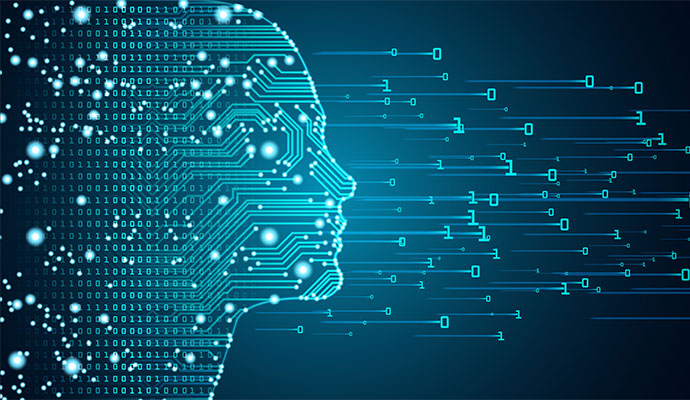### Software options and installation

There are many software packages that can be used to implement neural networks (NNs) for deep learning. This article compares the most popular as of 2019. We use TensorFlow. To install it on your computer, follow these instructions, which includes the step of installing Python. Be careful about versions. Python, pip and TensorFlow are being updated often. You will probably not want to run the very latest because they may not be compatible with each other. If you run into this problem, back up a few versions.

The Clemson CCIT group offers a series of training classes on python programming, big data, and machine learning. They also operate the Palmetto high performance computing cluster. Students can request an account on this platform to speed up computations or run them remotely.

### Simulations

The strength of deep learning is that it can be applied to complex classification problems, such as recognizing pictures of different types of animals (e.g. dogs vs cats). However, starting to study deep learning by working on these problems can be overwhelming. The purpose of these simulations is to enable students to study deep learning concepts on simple problems. Each problem has a specific known feature or challenge so that we can see how deep learning is identifying that feature.
• Simslope. Classifies 1D data that either trends downward (class 0) or upward (class 1). C code that generates random samples. Python code that uses TensorFlow to classify the samples. More python code that reads the TensorFlow model (generated by the previous python code) from a file and uses it to perform classification.

Lessons learned in this example include:

• How to normalize data. Note the original units are in the range 400...600. It needs to be in the range 0...1 for the gradient descent to work correctly. Try commenting out the normalization to see that the NN cannot learn the patterns.
• What can be done with a dense NN layer. It connects every input datum to every neuron. This example is essentially learning to template match.
• Understand the weight values learned in the NN. In this case, they range from positive through zero to negative for the downward slope class, and the opposite for the upward slope class. In effect the NN learned a template of downward vs upward slopes. Try running more iterations (1,000) to see how the patterns continue to become more clear in the learned weights.
• How to save a classifier model (training can take a long time) and then load it later for future classifications.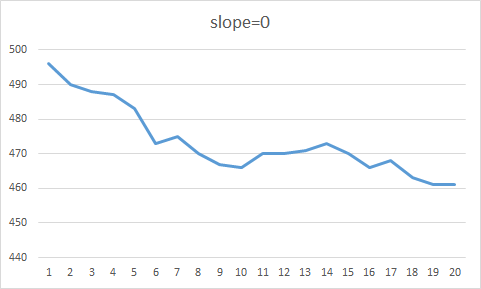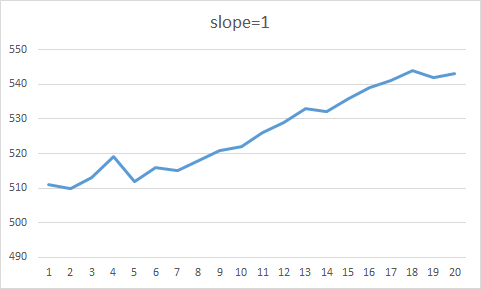• Simtrail. Classifies 1D data that resembles up-and-down trail hiking. Both classes have random periods of flat, up and down. Class 0 has shorter periods of flat after up, class 1 has longer periods of flat after up. The basic idea is that the classifier needs to use a convolutional layer to learn that the important feature is the length of a flat following an up. C code that generates random samples. Python code that uses TensorFlow to classify the samples.

Lessons learned in this example include:

• What can be done with a convolutional layer. It slides a window across all the data in a sample and learns local patterns. Compare this with a dense layer which learns global patterns. Try a dense layer to see that it cannot learn to classify this data.
• Parameters of a convolutional layer. The window needs to be long enough to capture the feature of interest. The number of patterns learned needs to be large enough so that the important one(s) are learned (e.g. it might learn patterns associated with just flat, just down, just up, and combinations of these). Experiment with changing the window size and number of features learned to see how this affects classification accuracy.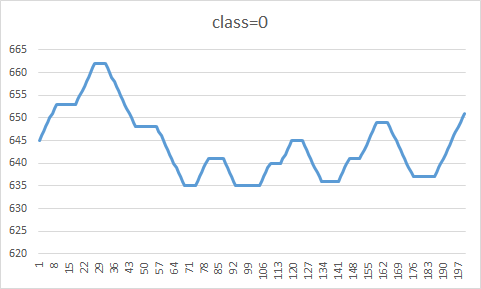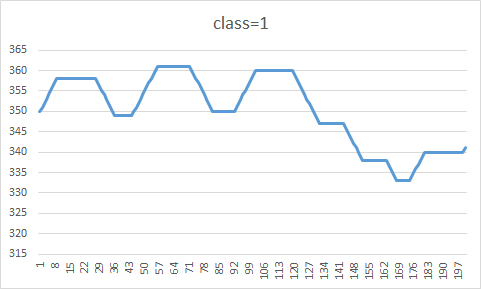• Sim6axes. Classifies 2D data captured from multiple sensors (resembles IMU data). Both classes have random periods of flat, up and down. For class 0, directional changes of the sensors are random and unrelated. For class 1, directional changes are correlated; if one sensor is going up then all six sensors will go up for the same period of time. The basic idea is that the classifier needs to use a 2D convolutional layer to learn that the important feature is the correlation of signal directions. C code that generates random samples. Python code that uses TensorFlow to classify the samples.

Lessons learned in this example include:

• What can be done with a 2D convolutional layer. It slides a window across multiple sensors of data to learn local patterns. Commonly used in image recognition but also applicable to IMU data. It should be noted that for image recognition, the convolutional layer is typically square, while for IMU data, the sensor dimension of the layer is typically much smaller than the time dimension. Try a 1D convolutional layer to see that it cannot learn to classify this data.
• Parameters of a 2D convolutional layer. As with the previous example, the window needs to be long enough to capture the feature of interest. The number of patterns learned needs to be large enough so that the important one(s) are learned. Experiment with changing the window size and number of features learned to see how this affects classification accuracy.• Simcontin. Classifies 1D data that has a continuous class label instead of having a small number (e.g. 2) of discrete classes (e.g. up or down). In this example the data for each sample is a line (with noise) and the class is the slope of the line. The basic idea is that the classifier needs to learn a continuous function rather than a discrete set of class labels. C code that generates random samples. Python code that uses TensorFlow to classify the samples.

Lessons learned in this example include:

• How to frame a problem that has a continuous classification label. The loss function needs to be changed. Read about different loss functions and try some.
• How to normalize data for this type of problem. Previous problems normalized each sample independently. This problem requires global normalization so that the feature differences between samples are not distorted. Experiment with changing the normalization to see how this affects classification accuracy.### Training on huge data sets

If a data set is too large to load into memory all at once then we can use batches to train on it incrementally. Each subset of the data is called a batch. The batch size can be tuned to balance against the number of epochs needed for training.

Batch training is programmed using python generators. The following python code examples serve as a tutorial to introduce generators and how to use them for batch training.

• Python code of a generator for the Fibonacci sequence.
• Python code for a generator that incrementally loads a subset of data from a single file. It uses simslope.txt as the data file.
• Python code for a generator that loads folds of data that are stored in separate files (incrementing through the filenames). It uses four data files: fold1.txt, fold2.txt, fold3.txt, fold4.txt.
• Python code for a generator that preprocesses data and returns a class label with each sample. It uses the 4 data files from the previous example.
• Python/TF code that trains using a generator. It uses all four folds of data during training.
• Python/TF code that trains using two generators, one for training data and one for validation data. It uses folds 1-3 for training and fold 4 for validation.

### Using a TensorFlow classifier in a C program

All TensorFlow models are trained in Python programs, but sometimes we want to deploy the classifier for use in a program in another language. In my group we write a lot of C programs. This section demonstrates how to use a TensorFlow model (classifier) in a C program.

First it is important to have some understanding of how a classifier is saved to a file. There are 3 important pieces: the graph, weights, and gradients. The graph defines the nodes and connections in the neural network. The weights are the values at the nodes used to make calculations. The gradients are used during training to continually update the weights. There are two ways to save a model: save everything so that training can be resumed, or ``freeze'' the model (omitting the gradients) so that the model can be used for classification but cannot continue training. There are a huge variety of file formats used in machine learning. For our purposes, we will use the H5 file format to store a complete model, and the PB file format to store a frozen model. Note that as of 2020 TensorFlow v2 has created a TF file format that tries to do both; it uses a folder to contain the basic PB file along with secondary files containing the checkpoint data (gradients) necessary to resume training.

• Download the C library for TensorFlow. Note that as of 2020 it is still stuck on TensorFlow v1.

Update: See this link for migrating from v1 to v2.

• This one-line C program tests linking to the C library for TensorFlow (to make sure your environment is set up correctly).
• This Python program demonstrates saving a frozen model in PB format after training. It uses the simslope data from an example above.
• This C program tests using the PB model saved from the previous bullet to perform classifications.
Each of those programs contains further info in the comments as well as links to other sites that explain more of the concepts.

### Tutorials from other sources

• A set of tutorial problems from the TensorFlow group. Click here to download the code for the classic MNIST problem which involves classifying images of the digits 0-9. The code downloads the data set for you.
• A blog by Nils Ackermann showing how to use deep learning to recognize human activities (e.g. walking, climbing stairs) from IMU data. A second blog by the same author extending the approach. Click here to download the code (excludes all visualization steps, just does classification). You will need to download the WISDM data set.

### Our data sets

In a set of projects we are using deep learning to study classification of datasets our group has collected over the years:
• Pedometer data set. Contains 3-axis accelerometer and 3-axis gyroscope data from devices worn on the wrist, hip and foot, while subjects walked. Classification problems include counting steps and determining gait type.
• Cafeteria data set. Contains 3-axis accelerometer and 3-axis gyroscope data from a device worn on the wrist, while subjects ate a meal. Classification problems include detecting intake gestures (bites) and classifying eating-related gestures (utensiling, drink, bite).
• Samsung data set. Contains images of washing machine parts during manufacturing and assembly. Classification problems include detecting when parts are not correctly installed. Note this data set is currently private to my research group.
• Eating detection data set. Contains 3-axis accelerometer, gyroscope and magnetometer data from a device worn on the wrist, while subjects were free-living all day. Classification problems include detecting periods of time when subjects were eating. Note this data set is currently private to my research group.